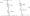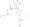# Electronic Circuits January 2008

Note ; Answer any FIVE full questions.

1 a.  Discuss voltage doubler circuit.

b.  Define regulation and derive equation for a fullwave circuit.

c.  In the Fig. 1(c) the diodes are ideal. Write the transfer characteristic equations (V0 Vs Vi). Plot V0 Vs Vi. Indicate all intercepts, slopes and voltage levels.Fig. 1(c)

Repeat for the case when Vr ~ 1 V Vj = 40 sin ot

2. a. Define three stability factors. For a self bias circuit derive the expression for Sv and S.

b.  For the circuit shown in Fig.2(b) Vcc = 24 V, Rc = 10 kO, Re = 270 Q, (3 = 45, Silicon transistor is used \mder operationing condition Vce – 5V find

i) R                                 ii) Stabiiityfector SFig.2(b)

c. Briefly discuss how do you provide operating point stability using compensation

technique.

3 a. Using small signal low frequency hybrid model for the CE amplifier with a  load of ZL and source resistance Rs derive expression for Ai, Ay, Z; and Z0 (10 Marks)

b.  For a transistor amplifier in CB configuration find Al3 Ay, Z,Ib Zout, Avs and AK given that hib = 22 Q, hfb = 2.9 x 10-4, h* = -0.98, l/h0b = 2.04 mQ.

c.  State and Prove Miller’s theorem.

4 a, Draw small signal high frequency CE model for transistor and explain the significance of even component in the model and prove that hfc = gm rb’e.

b.  Discuss the various types of distortion in amplifier.

c.  With suitable RC circuit calculate low frequency response of an amplifier.

5 a. Explain the advantages of negative feedback circuit.

b.  Draw and explain different feedback amplifier topologies.

c.  An amplifier with open loop voltage gain Av = 1000 ± 100 is available. It is necessary to have an amplifier whose voltage gain varies by no more than ± 0.1%.

i) Find the reverse transmission factor {3 of the feedback network

ii)  Find gain with feedback.

6 a. Show that the maximum conversion efficiency of class-B push pull amplifier is 78.5%.

b. Discuss why even harmonics are not present in push-pull amplifier.

c.  Explain:

i) Input bias current compensation in OP AMP.

ii)  Input offset voltage compensation for inverting and non inverting configuration.

7 a. Design a single pole LPF with-a cut off frequency of 10 kHz and mid band gain of 1.5. Draw the circuit diagram.

b.  With a neat diagram and relevant waveforms explain the following OP AMP circuits:

i) Peak detector and clamper

ii) Schmitt trigger

iii) Instrumentation amplifier

c. Explain sample and hold act.

8 a. With a neat diagram and relevant waveforms, explain the working of an a stable modulator

circuit using 555 timer. Obtain the expression for the time period.

b. Discuss the specifications of a DAC circuit.